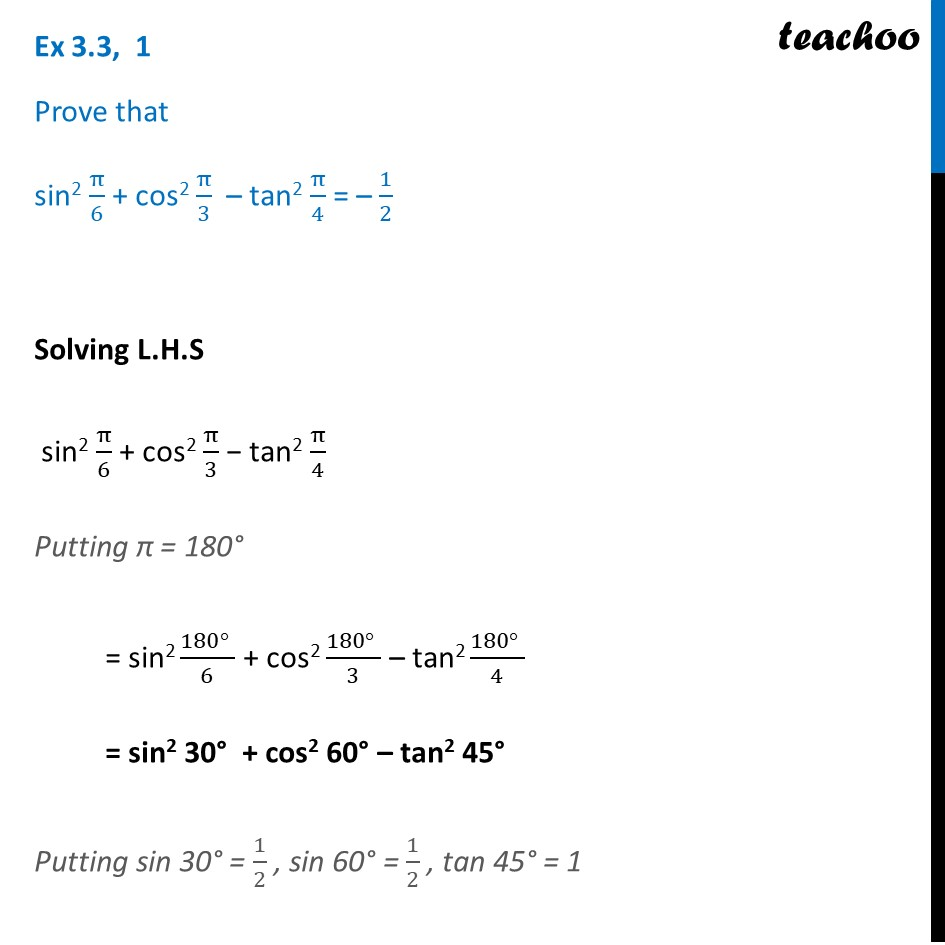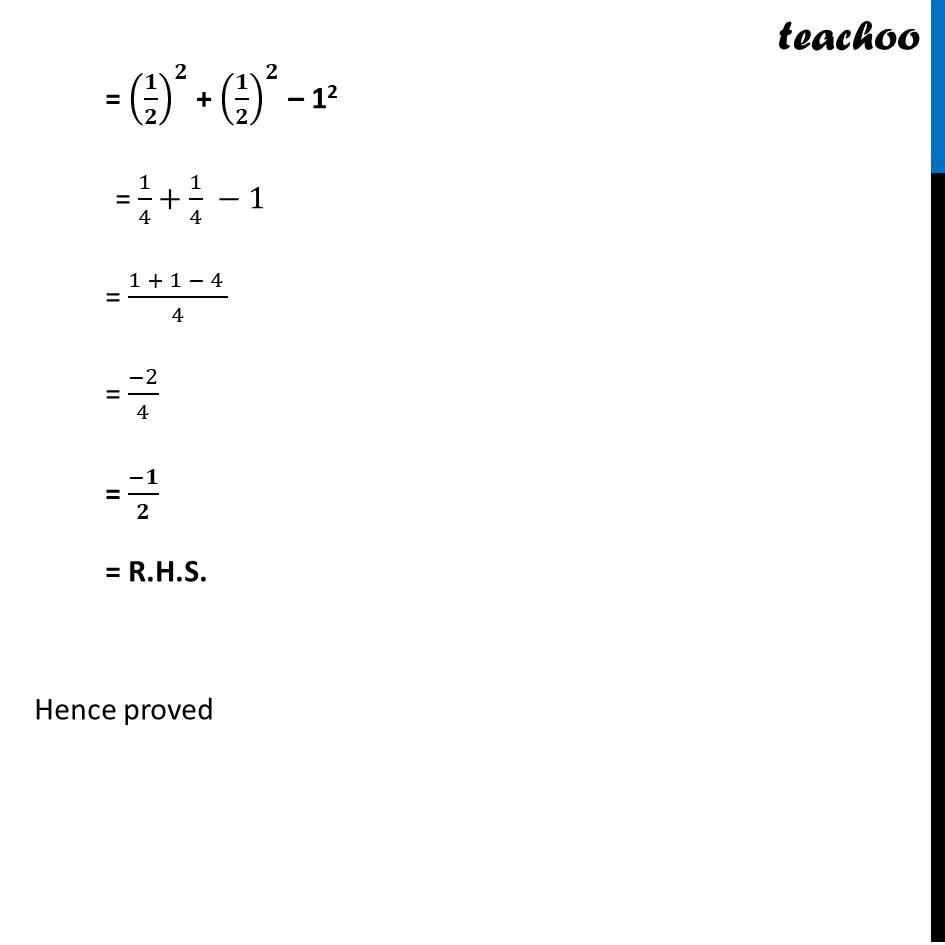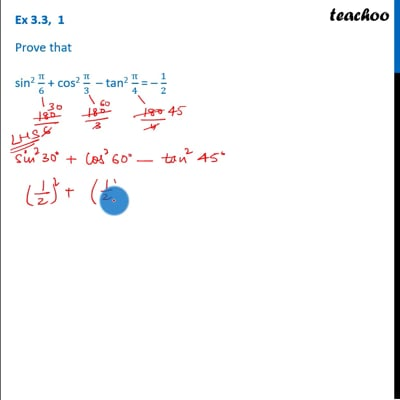Ex 3.3

Chapter 3 Class 11 Trigonometric Functions
Serial order wiseThis video is only available for Teachoo black usersThis video is only available for Teachoo black users

Get live Maths 1-on-1 Classs - Class 6 to 12

### Transcript

Ex 3.3, 1 Prove that sin2 π/6 + cos2 π/3 – tan2 π/4 = – 1/2 Solving L.H.S sin2 π/6 + cos2 π/3 − tan2 π/4 Putting π = 180° = sin2 (180° )/6 + cos2 (180° )/3 – tan2 (180° )/4 = sin2 30° + cos2 60° – tan2 45° Putting sin 30° = 1/2 , sin 60° = 1/2 , tan 45° = 1 Ex 3.3, 1 Prove that sin2 π/6 + cos2 π/3 – tan2 π/4 = – 1/2 Solving L.H.S sin2 π/6 + cos2 π/3 − tan2 π/4 Putting π = 180° = sin2 (180° )/6 + cos2 (180° )/3 – tan2 (180° )/4 = sin2 30° + cos2 60° – tan2 45° Putting sin 30° = 1/2 , sin 60° = 1/2 , tan 45° = 1 = (𝟏/𝟐)^𝟐 + (𝟏/𝟐)^𝟐 – 12 = 1/4+ 1/4 −1 = (1 + 1 − 4 )/4 = (−2)/4 = (−𝟏)/𝟐 = R.H.S. Hence proved